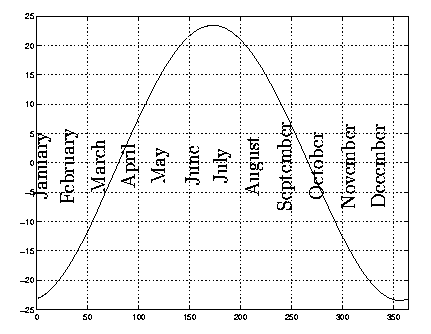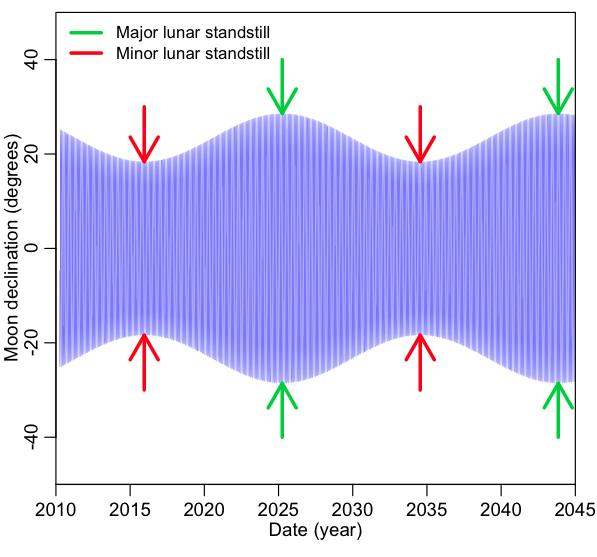Archaeoastronomy: where on the horizon do the stars, Sun, Moon rise and set? (Part I)

[This post is part of a series of posts on archaeoastronomy using open source software]

In astronomy, the observer’s coordinate system for observing a star (or the Sun or Moon) involves two coordinates, azimuth and altitude.Altitude is the angle from horizontal horizon measured from -90 to 90 degrees, where 0 is the horizon, and negative numbers mean that the body is below the horizon, and azimuth is from 0 to 360 measured from N.  This coordinate system is known as the “horizontal” coordinate system.

Is it obvious to you that when a star is rising or setting, the altitude is zero?

From our point of view, we are standing still, and the Sun/Moon/stars move across the sky.  But from a star’s point of view, equatorial coordinates make more sense, where we project the Earth’s pole and equator onto the celestial sphere:In this coordinate system, the star has two coordinates, the declination (kind of like latitude), and the right ascension (kind of like longitude).  It turns out that calculating the rise/set azimuth of a star only depends on the observer’s latitude on Earth, and the declination of the star.  If you wanted to calculate when the star would rise and set on a particular day, you would also need both the observer’s longitude on Earth, and the star’s right ascension.

However, regardless of what time of day the star rises or sets, to a good approximation, over our lifetimes at a particular location, the star always rises and sets at exactly the same azimuths on the horizon (as we’ll discuss later, the declinations of stars tend to slowly move over the centuries for a number of reasons, but the movement is so slow as to be imperceptible to the human observer over a lifetime).  Thus, for assessing whether or not an archaeological site has alignments to the rise/set of a particular star, all we need to know is the star’s declination at the year the site was built, and the latitude of the site.

You can find catalogs of the declinations of the brightest stars all over the internet.  Here is an example of one.  Notice that the catalog includes the measurements of something called the visual magnitude (“V Mag”… aka apparent magnitude).  This is a measure of the brightness of a star.  The smaller the number, the brighter the star.  Humans on a dark night can generally see stars down to about magnitude 6.  The names of many of the brightest stars (Sirius, Arcturus, Vega, Betelgeuse, etc) are familiar to many of us, even if we aren’t interested in star-gazing enough to necessarily know exactly where they are in the night sky.  Here is an example of the nighttime sky in spring in the Northern Hemisphere:The US Naval Observatory has a nice online calculator for the rise/set azimuths of celestial bodies.  Let’s use it to compute the rise/set azimuth for the star Sirius in Chicago for today.  I get that it will rise at azimuth 112 degrees, and set at azimuth 248 degrees.  Do you think these numbers will change with the date?  Try it out.  What result does change when you change the date?

How is the US Naval Observatory calculating the rise/set azimuths?  They convert between the equatorial to the horizontal coordinate system. Converting between these two coordinate systems requires some geometrical gymnastics.  But it turns out there is a simple formula for calculating the rise/set azimuth, A, given the declination, delta, and latitude, phi.  It is

cos(A)= sin(delta)/cos(phi)

Now, the latitude of Chicago is phi=41.85N, and the declination of Sirius is delta=-16.71611 degrees.  Convert these numbers to radians (multiply by pi and divide by 180) and plug them into the formula above.  I get that A at rise/set is +112.7 or -112.7 (ie; 112.7  or 247.2).  These are indeed quite close to the numbers calculated by the US Naval Observatory.

What about the rise/set azimuths of the Sun and Moon?

Unlike the stars, the declination of the Sun and Moon are constantly changing over time.  For the Sun, the changes have a periodic pattern over an annual cycle, and change from a declination of -23.5 degrees at winter solstice to +23.5 degrees at summer solstice (phi=23.5 degrees is currently the tilt of the Earth’s rotational axis with respect to the plane of its orbit around the Sun).For the Moon, there is a 29.5 day (on average) periodic pattern in the declination linked to the phases of the Moon (known as the synodic or lunar period). There is also a much longer periodic pattern known as the Saros Cycle, and it has period 18.6 years (due to the fact that the plane of the Moon’s orbit is actually tilted 5.1 degrees with respect to the Earth’s equator).  The resulting plot of the declination of the Moon over time looks like this:The maximum declination the Moon can achieve is (23.5+5.1)= 28.6 degrees.  This is known as a major lunar standstill. There is another special point 18.6/2 years later where the maximum declination that can be achieved is (23.5-5.1)=18.4 degrees.  This is known as a minor lunar standstill.  The next minor lunar standstill will occur in Fall of 2015, and the next major lunar standstill will occur in Spring of 2025.

As far as archaeoastronomy is concerned, the usual assumption is that an archaeological site would have alignments to the Sun winter and summer solstice, and/or the major and minor lunar standstills.

Tiddly things

There are a few (usually) minor corrections that need to be taken into account in order to accurately determine the rise/set azimuth of a star/Sun/Moon at a particular location.  One is the fact that the atmosphere refracts light, and acts something like a lens.  Because of this, we can see the Sun/Moon/stars apparently at the horizon even when the altitude calculated from equatorial/horizontal coordinate conversion is actually still negative:The correction needed to the altitude is approximately half a degree.  In the geometrical gymnastics that we do to convert from the equatorial coordinate system to the horizontal coordinate system, we can correct for refraction by finding the azimuth that yields an altitude angle equal to negative of the refraction angle.

In addition, the Sun and the Moon are not point sources of light.  Their discs both subtend an arc about half a degree (they just happen to be about the same size from our perspective, which is why during a solar eclipse the Moon almost exactly eclipses the Sun… half a degree of arc btw is approximately the angle subtended by an aspirin held at arms length). In our society, “sunrise” and “moonrise” are defined by when the top of the disc of the Sun and Moon are just visible at the horizon.  The center of the Sun and Moon are below the horizon at that point, so in the calculation of the azimuth of the rise, we need to correct the dip angle for the width of the disc.  One thing I’ve found curious is that in archaeoastronomy it seems to be universally assumed that ancient societies defined sunrise and moonrise the same way we do… if instead “moonrise” is defined as the point when the entire disc of the Moon has lifted above the horizon, then that can potentially change the azimuth of the rise by a non-negligible amount.

A little bit trickier is the correction for the true horizon.  Unless we are at sea, or on a flatter-than-a-pancake prairie, our visual horizon is not the flat horizon.  There are mountains and valleys.  The presence of a mountain can substantially change the observed rise azimuth of a celestial body:Notice that the star in the above figure is not rising perpendicular to the horizon; there is the added complication in this calculation that at most places on Earth the stars/Sun/Moon don’t rise perpendicular to the horizon. Because of the Earth’s tilt they actually rise at an angle to the horizon:The angle between the planes of the two circles (the observers horizon, and the path of the star on the celestial sphere) is 90-phi, where phi is the latitude.  The slope of the angle the star trajectory makes with the horizon as it rises is, to a good approximation,

a = sqrt(cos(latitude)^2-sin(declination)^2)/sin(latitude)

(thus it is only at the equator that this slope goes to infinity, and thus the Sun/Moon/stars rise perpendicular to the horizon). The slope of the angle the star trajectory makes with the horizon as it sets is -a.

To determine the true rise azimuths of stars at a particular location on Earth, you would need to obtain a survey of the horizon altitude vs azimuth.  Then, based on the star’s declination and the observer latitude, we can calculate the altitude/azimuth trajectory of the star across the sky.  Then we look for the altitude/azimuth along that trajectory that intersects with the horizon altitude/azimuth.

In the R file archeoastronomy_libs.R I provide a function calculation_rise_set_azimuths(). Given an observers latitude, and the declinations of stars/Sun/Moon, the script calculates the rise and set azimuths for a flat horizon (assuming our societal definition of Sun and Moon rise), correcting for refraction and the size of the Sun/Moon disc. The function also has the options where the user can supply a vector, horizon_altitudes, of horizon altitude measurements taken at azimuths, horizon_azimuths.  If these are given, then the function calculates the star/Sun/Moon rise and set azimuths with respect to that horizon.

In the next module, we’ll discuss how to get the horizon profile using data from the US Geological Survey website.